# Arithmetic

#### Simple Interest & Compound Interest - 1

Time Speed and Distance - 1

Circular MotionThe concept of circular motion is an extension of the chapter time, speed and distance. It involves the same concepts of relative speeds, length of the track, time taken but applied in a different scenario. Circular motion involves sprinters and runners running along a circular path. They might be running in the clockwise or the anticlockwise direction. In a circular path there is a starting point but not a terminating point, the starting point could act as the terminating point. One by one let’s discuss on the various aspects of this topic.

Let’s say there are two runners A and B running in the same direction starting from the same point with speeds of 5m/s and 8m/s and  length of the track is 40m.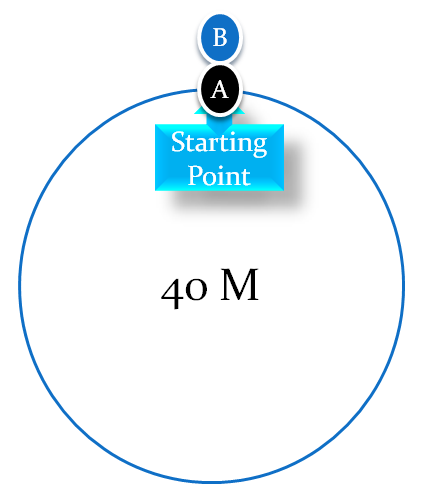Now, A can complete one lap in (40/5) = 8 seconds and B can complete the same lap in (40/8) = 5 seconds. We can say that A reaches the starting point at every 8 seconds while B reaches the starting point at every 5 seconds. If we were to find out the time both the runners will be together at the starting if they run non-stop, then ,

A reaches the starting point at 8,16,24,32,40,48,56,………..seconds

B reaches the starting point at 5,10,15,20,25,30,35,40,45,50,…..seconds

Thus, they are together at the starting point at 40 seconds for the first time and again will be at the starting point at every multiple of 40 thereafter.

Here 40 seconds is the L.C.M. of (8,5).

What if the runners were running in the opposite direction? The answer would remain the same because it is independent of the direction in which the runners are running.

In general we can say if the speeds of the runners are v1 and v2 while the track length is L, then the time in which they will meet for the first time at the starting point is L.C.M. of (L/v1 , L/v2) .

Now, we shall find the time at which the runners will meet for the first time. Here, there are two cases:

Case1:  Running in the opposite direction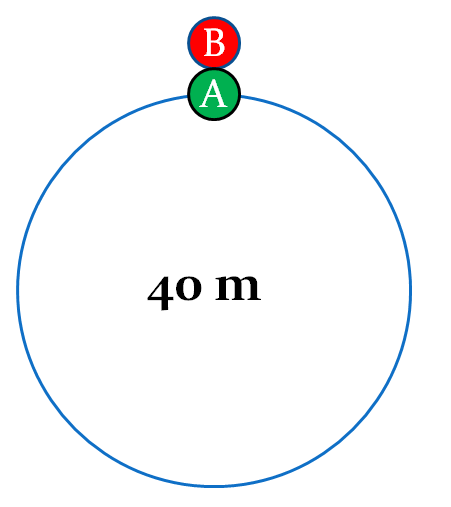Let’s say the two runners are running in the opposite directions with speeds of 3m/s and 5m/s respectively in a circular track of length 40m. If we have to find out the first instant they meet each other, then how to proceed?

If the runners are running in the opposite direction, then their relative speed is 3+5=8 m/s

Time taken to meet each other will be 40/8 = 5 seconds. Thus, after every 5 seconds they will meet each other.

Also, we can say that as the time is constant, so distance is directly proportional to speed. Thus, distances travelled by them will also be in the ratio of their speeds, i.e. 3:5. Thus, the  first runner would have travelled 15m and the other 25m and together they would have completed one lap.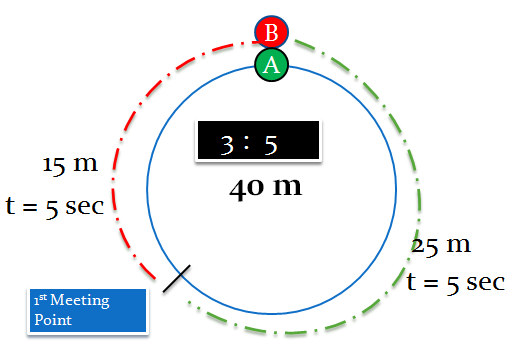Let’s say the runners continue running after their first meet. Again they will meet after 5 seconds and the distances travelled by them will 15m and 25m as shown in the figure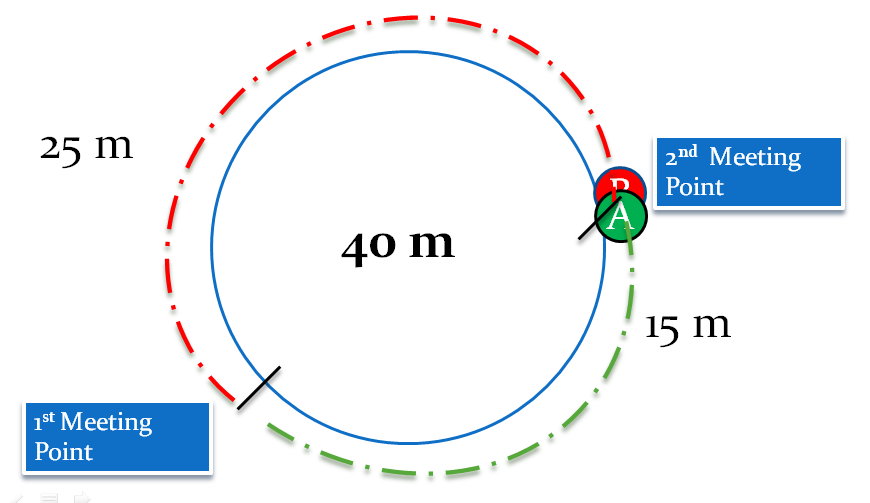Again they have completed one lap together. So, we can say that every time they meet each other, they would have collectively travelled one lap.

Also, in every meet they would travel the same distance if their speeds remain constant.

So, by this logic we can say that the sum of the number of laps completed by the two runners will tell us the number of times they have met during a given time frame when running in the opposite directions.

Case2:  Running in the same direction:

Let’s say the two runners A and B are running in the same direction with speeds of 2m/s and 5 m/s respectively and the length of the track is 60 m.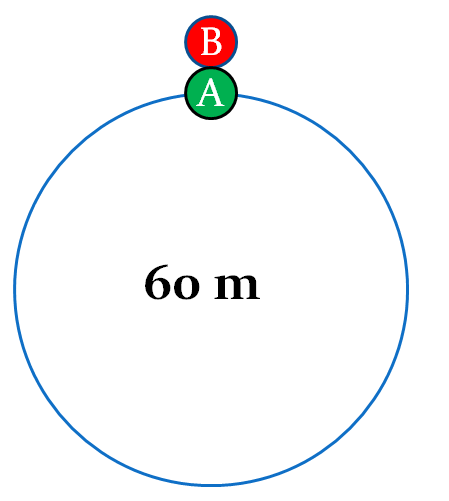As the two runners are running in the same direction, their relative speed will be

5-2 = 3m/s.

Now we can say that time taken by B to meet A = 60/3 = 20 seconds.

And after every 20 seconds they will meet each other.

Distance travelled by A in 20 seconds = 2*20 = 40 m and the distance travelled by B = 20*5 = 100m

This distance travelled by B is 40 + 60 i.e. B has completed one extra round compared to A.

And this would happen every time the two runners will meet each other, i.e. they would meet after 20 seconds and B would travel an extra round compared to A.

So, by this logic we can say that the difference in the number of laps completed by the two runners will tell us the number of times they have met during a given time frame when running in the same direction.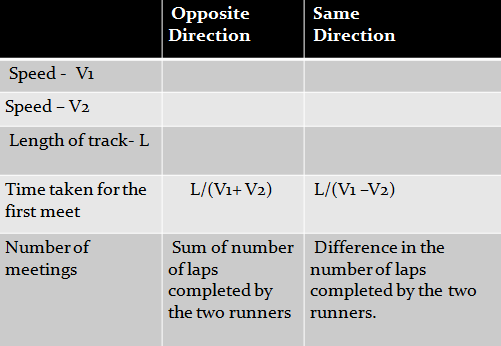Example 1: Three runners A, B and C are running along a circular track of length 120 m with speeds of 18km/hr, 27km/hr and 72km/hr in the same direction.

a. At what first instant will all of them be together at the starting point?

b. At what first instant will all of them be together?

Solution: First we shall convert their speed in to m/s

Speed of A = 18 * (5/18) = 5m/s

Speed of B = 27 * (5/18) = 7.5 m/s

Speed of C = 72 * (5/18) = 20m/s

a. Time taken by A to complete one lap = 120/5 = 24seconds

Time taken by B to complete one lap = 120/7.5 = 16 seconds

Time taken by C to complete one lap = 120/20 = 6 seconds

The first instant at which all the three would be at the starting point = L.C.M.(24,16,6) = 48 seconds

b. Relative speed of A and B = 7.5 -5 = 2.5 (Running in the same direction)

So, time taken by A to meet B = 120/2.5 = 48 seconds

Relative speed of A and C = 20 – 5 = 15 (Running in the same direction)

So, time taken by A to meet C = 120/15 = 8 seconds

The first instant at which all the three would be together = L.C.M.(48,8) = 48 seconds.

Example 2: P can run one full round of a circular track in 4 min while Q can run  the same track in 7 min. Both P and Q start simultaneously from the same point. How many times would they meet each other when running in the same direction and in the opposite direction if Q has completed 12 rounds.

Solution: The ratio of time taken by P and Q to complete one round is 4:7

So, their speeds are in the inverse ratio of their times taken i.e. 7:4

When they meet each other time is constant

So, distance travelled is in the same ratio as their speeds

So rounds completed by them is also in the ratio of 7:4

If Q has completed 12 rounds, then number of rounds completed by P = (7/4)*12

=21

Number of meetings when running in the same direction = 21-12 =9

Number of meetings when running in the opposite direction = 21+12 = 33

Example3 : Two sprinters are running in the clockwise direction along a circular track of length 6km. The faster sprinter overtakes the slower one every two hours. If the faster sprinter completes one lap six minutes sooner than the slower one, then, what is the speed of the slower sprinter?Example 4: Tom and Jerry are running along a circular track Starting from the same point in the same direction. By the time they met for the 10th time, how much more distance than Jerry would have Tom travelled, if the speeds of Tom and Jerry are 12m/s and 10m/s and the radius of the track is 14m ?

Solution: Given radius of the track = 14m

So, length of the track = 2πr = 2*(22/7)*14 = 88m

We know that whenever, the faster runner completes one extra round compared to the slower runner, they meet each other

Number of times they have met = 10

Number of extra laps taken by Tom = 10

Extra distance covered by Tom compared to Jerry = 10* 88 = 880 m

Example 5: Sunny and Nitish start running from a point A on a circular track with different speeds and in opposite directions. Sunny runs in the clockwise direction and meets Nitish at a distance of 700 m in the clockwise direction for A. They again meet each other for the second time at a distance of 600 m in the anticlockwise direction from A. If Nitish is still in his first round, then what is length of the track?

Solution: As the two runners are running in the opposite direction, the sum of the distances travelled by them in the first meet would be equal to the length of the track. Let the length of the track be L and distance travelled by Nitish is ‘x’.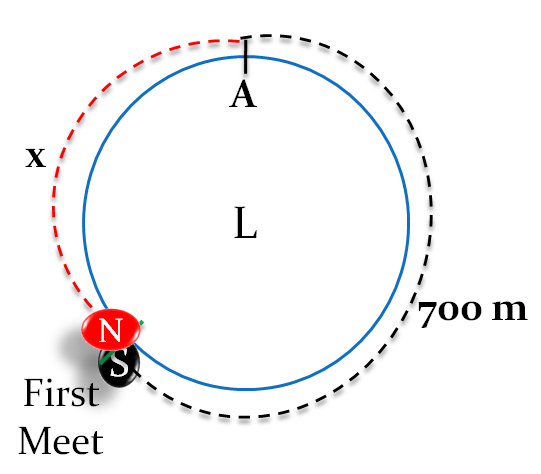So, we can say that L = x+700  (1)

In the next meet again, both of them would have travelled the same distance, i.e. Nitish would have covered x metres and Sunny 700 metres.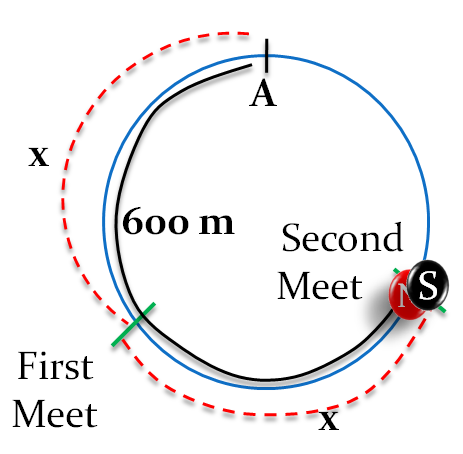From the figure it is clear that 600 = x+ x or  x = 300m

Thus, length of the track is L = 700 + x   (From (1) )

= 700 + 300 =1000m

Distinct Meeting Points:

If we are the figure out the number of distinct meeting points on the track where the two runners will meet each other, then there are again two cases – running in the opposite direction and in the same direction.

Running in the opposite direction:

Let’s say there are two runners starting from the same point and at the same time running in the opposite directions with speeds of 2m/s and 3m/s on a track of length 5m.

In their first meet, they would have covered distances in the ratio of 2:3 (same as their speeds), so distances covered by them become 2m and 3m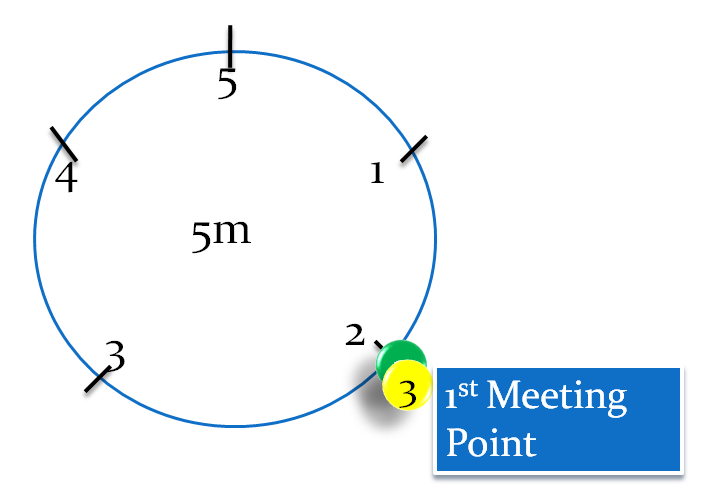They continue moving and again during their second meet they would have covered 2m and 3m respectively.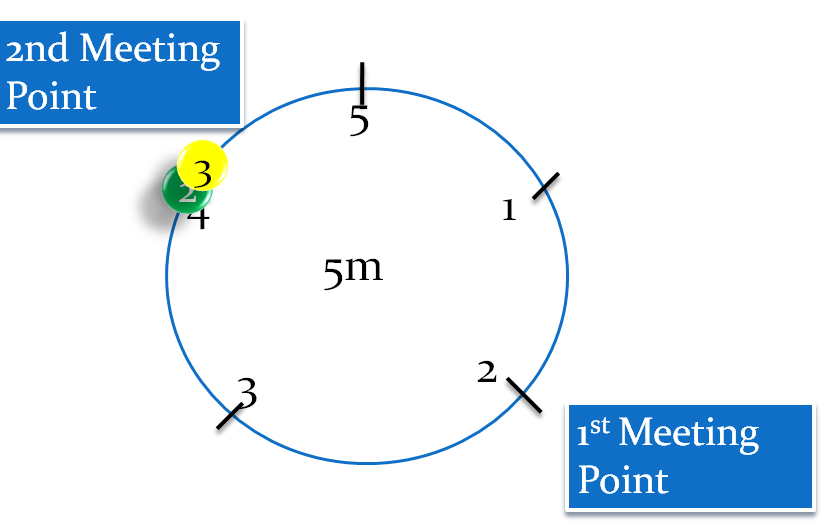Continuing on the same logic, we get the following distinct meeting points on the track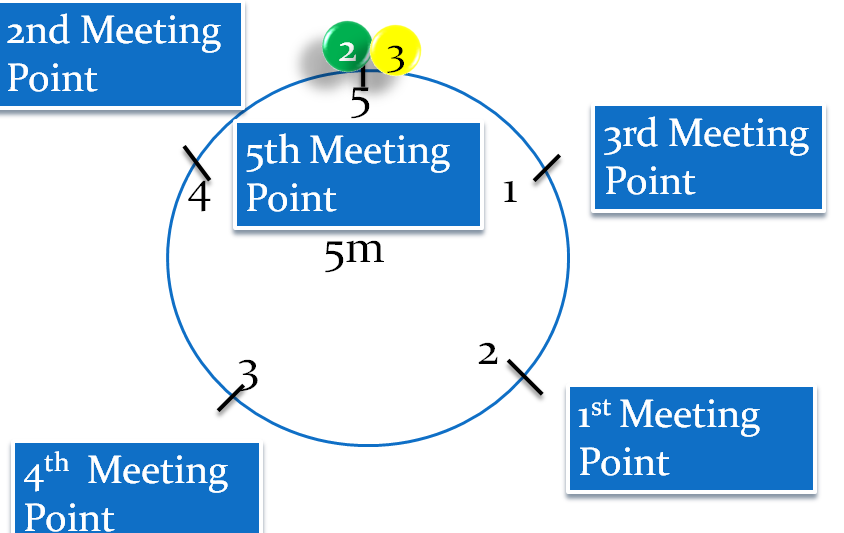Thus, these are the five distinct points on the track where the two runners will meet each other and in fact if they continue running then they will be meeting at only these points.

Now the speeds were in the ratio of 2:3 and distinct points were 2+3 = 5

So, we can say that if the speeds of the runners are in the ratio of a:b, then distinct points on the track where they meet are

1. ( a+b) – When running in the same direction.

2.  (a –b)  - When running in the opposite direction.

Note: Here the ratio of speeds must be in their simplest form. For example if the speeds of two runners are 6 and 10 m/s, then first we need to reduce them to their simplest form i.e 3:5 and then the number of distinct points will be 3+5 = 8 when running in the opposite direction and 5-3 = 2 when running in the same direction.

Example 6: Six runners A,B,C,D,E,F have speeds in the ratio of 1:2:3:4:5:6. A,C, and E are running in the clockwise direction, while B,D and F in the anticlockwise direction starting from the same point. In how many distinct points on the track, do at least  any of the two runners meet each other?

Solution: The ratio of speeds of A,B,C,D,E and F are 1:2:3:4:5:6 respectively.

If we consider the runners A and B, then they are running in the opposite directions and the ratio of their speeds is 1:2. So, number of distinct points = 1+2 = 3

So, we divide the track length into three equal parts. Thus, their points of meeting become: L/3, 2L/3 and L

If we consider A and C, then they are running in the same direction. So, number of distinct points become: 3-1 =2

So, we will divide track length into two equal parts, i.e. L/2 and L.

Similarly the different meeting points for any pair of runners are calculated as below: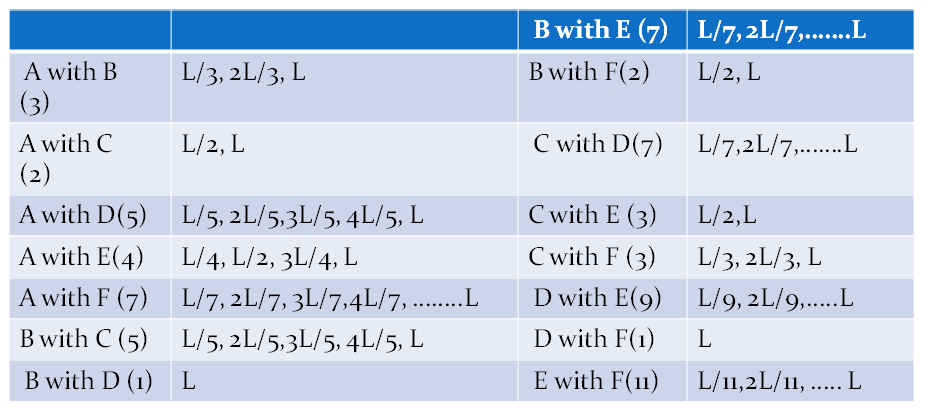Thus, there are 32 such distinct meeting points on the track.

Example 7: Two cars A and B start simultaneously on a circular track of length of 8km from a point O in the opposite directions.  Every time they meet each other, A increases its speed by 2km/hr while B decreases its speed by 2km/hr. If they met for the 5th time at 4pm and for the 19th time at 4:56 pm, then at what time did they meet for the 8th time?

Solution: The length of the track is 8km and the runners are running in the opposite directions. Also, whenever they meet, A increases its speed by 2km/hr while B decreases by 2km/hr

This means the relative speed of both A and B remains the same (As the speeds get added when running in the opposite direction)

So, time taken for each meet remains the same ( As Time = Length of track/Relative Speed)

Now time interval between 5th and 19th meet = 4:56 -4:00 = 56 min

Also number of times they have met in this interval = 14

Time interval between each meet = 56/14 =4 min

Now, we need to find the time at which they met for the 8th time.

There are three more meetings(6th, 7th and 8th)

So, time taken in these meetings = 3*4 = 12 minutes

So, the two runners would have met each other at 4:12 pm

Example 8: A sprinter starts running on a circular path of radius r meters. Her average speed (in metres/minute) is πr during the first 30 seconds.πr/2 during the next one minute, πr/4 during next 2 minutes, πr/8 during next 4 minutes and so on. What is the ratio of the time taken for the nth round to that for the previous round? (CAT 2004)

A. 4                    B. 8             C. 16                  D. 32

Solution: Here the speed of the sprinter is changing with respect to time.

Distance travelled by the sprinter during the first 30 seconds = πr*(1/2) = πr/2

Distance travelled by the sprinter during the next 1 minute = πr/2 * 1 = πr/2

Distance travelled by the sprinter during the next 2 minutes = πr/4 *2 = πr/2

Distance travelled by the sprinter during the next 4 minutes = πr/8*4 = πr/2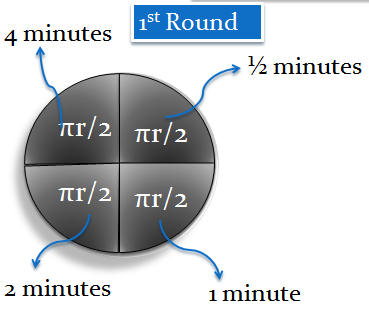So, by now the sprinter has completed one round and the time taken to complete the first round = ½ + 1 + 2 + 4 = 15/2 minutes

Similarly the time taken for the sprinter to complete the second round = 8+16+32+64 = 120 minutes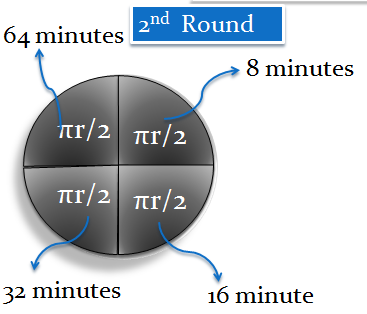Thus, ratio of time taken for the second round to the first round = 120/(15/2) = 16 ,which is the same as the ratio of time taken for the sprinter for the nth round to that for the previous round.